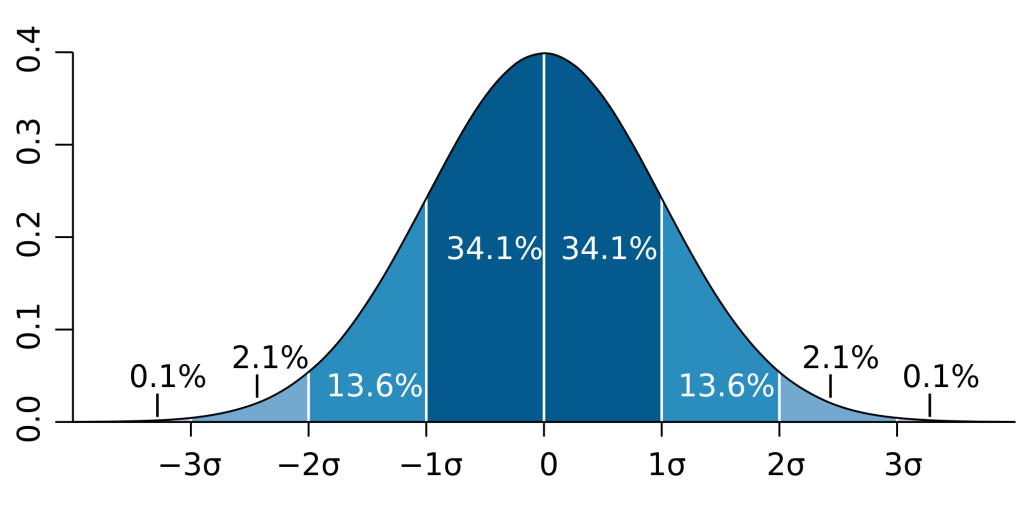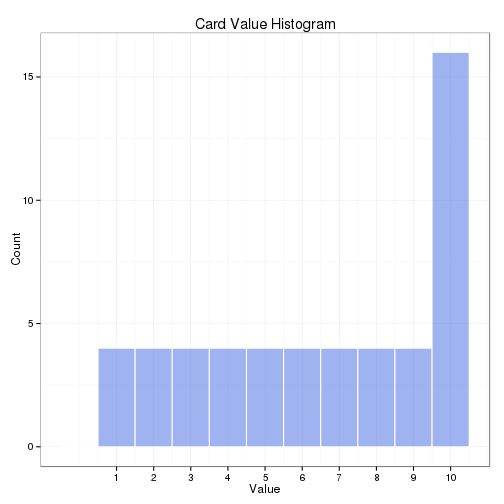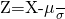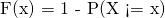Want to share your content on R-bloggers? click here if you have a blog, or here if you don't.• Source

#### Overview

Welcome to the Descriptive Statistics Final Project! In this project, you will demonstrate what you have learned in this course by conducting an experiment dealing with drawing from a deck of playing cards and creating a writeup containing your findings. Be sure to check through the project rubric to self-assess and share with others who will give you feedback.

#### Questions for Investigation

This experiment will require the use of a standard deck of playing cards. This is a deck of fifty-two cards divided into four suits (spades (♠), hearts (), diamonds (), and clubs (♣)), each suit containing thirteen cards (Ace, numbers 2-10, and face cards Jack, Queen, and King). You can use either a physical deck of cards for this experiment or you may use a virtual deck of cards such as that found on random.org (http://www.random.org/playing-cards/). For the purposes of this task, assign each card a value: The Ace takes a value of 1, numbered cards take the value printed on the card, and the Jack, Queen, and King each take a value of 10.

1. First, create a histogram depicting the relative frequencies of the card values.
2. Now, we will get samples for a new distribution. To obtain a single sample, shuffle your deck of cards and draw three cards from it. (You will be sampling from the deck without replacement.) Record the cards that you have drawn and the sum of the three cards’ values. Repeat this sampling procedure a total of at least thirty times.
3. Let’s take a look at the distribution of the card sums. Report descriptive statistics for the samples you have drawn. Include at least two measures of central tendency and two measures of variability.
4. Create a histogram of the sampled card sums you have recorded. Compare its shape to that of the original distribution. How are they different, and can you explain why this is the case?
5. Make some estimates about values you will get on future draws. Within what range will you expect approximately 90% of your draw values to fall? What is the approximate probability that you will get a draw value of at least 20? Make sure you justify how you obtained your values.

library(ggplot2)

### Create card deck

suits <- c("D", "C", "H", "S")
cards <- c("A", as.character(seq(2,10)), "J", "Q", "K")
values <- c(1, 2:9, rep(10, 4))

# Build deck
deck <- expand.grid(cards=cards, suits=suits)
deck$value <- values deck ## cards suits value ## 1 A D 1 ## 2 2 D 2 ## 3 3 D 3 ## 4 4 D 4 ## 5 5 D 5 ## 6 6 D 6 ## 7 7 D 7 ## 8 8 D 8 ## 9 9 D 9 ## 10 10 D 10 ## 11 J D 10 ## 12 Q D 10 ## 13 K D 10 ## 14 A C 1 ## 15 2 C 2 ## 16 3 C 3 ## 17 4 C 4 ## 18 5 C 5 ## 19 6 C 6 ## 20 7 C 7 ## 21 8 C 8 ## 22 9 C 9 ## 23 10 C 10 ## 24 J C 10 ## 25 Q C 10 ## 26 K C 10 ## 27 A H 1 ## 28 2 H 2 ## 29 3 H 3 ## 30 4 H 4 ## 31 5 H 5 ## 32 6 H 6 ## 33 7 H 7 ## 34 8 H 8 ## 35 9 H 9 ## 36 10 H 10 ## 37 J H 10 ## 38 Q H 10 ## 39 K H 10 ## 40 A S 1 ## 41 2 S 2 ## 42 3 S 3 ## 43 4 S 4 ## 44 5 S 5 ## 45 6 S 6 ## 46 7 S 7 ## 47 8 S 8 ## 48 9 S 9 ## 49 10 S 10 ## 50 J S 10 ## 51 Q S 10 ## 52 K S 10 ### 1. Create a histogram depicting the relative frequencies of the card values First, create a histogram depicting the relative frequencies of the card values. ggplot(deck, aes(x=values)) + geom_histogram(binwidth=1, origin=-0.5, col="white", fill="royalblue", alpha=0.5) + labs(x="Value", y="Count", title="Card Value Histogram") + scale_x_continuous(breaks = seq(1,10)) + theme_bw()### 2. Get samples for a new distribution Now, we will get samples for a new distribution. To obtain a single sample, shuffle your deck of cards and draw three cards from it. (You will be sampling from the deck without replacement.) Record the cards that you have drawn and the sum of the three cards’ values. Repeat this sampling procedure a total of at least thirty times. df <- c() for (i in 1:5000) { df$value[i] <- sum(sample(deck$value,3,replace = FALSE)) } samples <- data.frame(df) ### 3. Report descriptive statistics for the samples Let’s take a look at the distribution of the card sums. Report descriptive statistics for the samples you have drawn. Include at least two measures of central tendency and two measures of variability. summary(samples) ## value ## Min. : 3.00 ## 1st Qu.:16.00 ## Median :20.00 ## Mean :19.55 ## 3rd Qu.:23.00 ## Max. :30.00 ### 4. Create a histogram of the sampled card sums you have recorded Create a histogram of the sampled card sums you have recorded. Compare its shape to that of the original distribution. How are they different, and can you explain why this is the case? ggplot(samples, aes(x=value)) + geom_histogram(binwidth=1, origin=-0.5, col="white", fill="royalblue", alpha=0.5) + labs(x="Value", y="Count", title="3 Card Sum Histogram (n=5000)") + theme_bw()### 5. Make some estimates about values you will get on future draws Make some estimates about values you will get on future draws. Within what range will you expect approximately 90% of your draw values to fall? What is the approximate probability that you will get a draw value of at least 20? Make sure you justify how you obtained your values. #### a. Within what range will you expect approximately 90% of your draw values to fall? quantile(samples$value, probs = seq(.05, .95, 0.9))
##  5% 95%
##  11  28

#### b. Approximate probability that you will get a draw value of at least 20?

Calculate the z scoreatleast <- 20
mean <- mean(samples$value) sd <- sd(samples$value)
z <- (atleast-mean)/sd
z

##  0.08467613

We could lookup the value in the Z score table, but I want to calculate it.

Convert the Z score to a p-value with the Cumulative Distribution Function. We want to find the probability is larger than the given number so we use the lower.tail=FALSE.cdf <- pnorm(z, lower.tail=FALSE)
cdf

##  0.4662594

As we can see the probability that we will get a draw value of at least 20 is 0.4662594.

The post Descriptive Statistics Final Project with Python & R appeared first on Networkx.